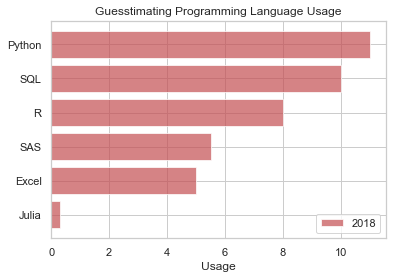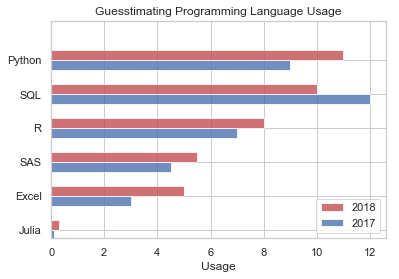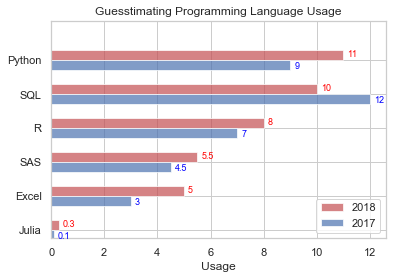# Horizontal Bar Chart: A stroll through the languages of data¶

#### Some preliminaries¶

If you are wondering why in the world this webpage looks the way it does, it might help you to review Anaconda, Jupyter scripts and a basic Python example. You can do so by reviewing the post(s) below.

### Using Matplotlib¶

Trying to figure out which predictive modeling programming language is the "best" is a bit like the Greatest Of All Time (GOAT) debate between who is better, Federer or Nadal?* Interesting but ultimately, useless. People use or prefer different languages for a myriad of reasons. We won't get into any of that, but will use some data around the popularity of various languages in order to showcase a few bar-charting capabilities in Python!

The data we use is (very loosely) based on the following posts: http://r4stats.com/articles/popularity/ | https://www.kdnuggets.com/2018/05/poll-tools-analytics-data-science-machine-learning-results.html

We start our foray into horizontal bar charts using the popular Matplotlib library (https://matplotlib.org/). This was inspired from MATLAB, and provides a lot of control over nearly every aspect of the chart (at the cost of lots of coding!).

*silly question: Nadal of course! :)

In :
import numpy as np
import seaborn as sns
sns.set(style="whitegrid") #makes the graph look a little nicer
import matplotlib.pyplot as plt #we load the library that contains the plotting capabilities
from operator import itemgetter #we use this in the sorting procecure, below

D = [('SQL',10),('Python',11),('R',8),('SAS',5.5),('Julia',0.3),('Excel',5)] #enter data for language & popularity
Dsort = sorted(D, key=itemgetter(1), reverse=False) #sort the list in order of popularity

lang = [x for x in Dsort] #create a list from the first dimension of data
use  = [x for x in Dsort] #create a list from the second dimension of data

plt.barh(lang, use, align='center', alpha=0.7, color='r', label='2018') #a horizontal bar chart (use .bar instead of .barh for vertical)
plt.yticks(lang)
plt.xlabel('Usage')
plt.title('Guesstimating Programming Language Usage')
plt.legend() #puts the year, e.g. 2018, on the plot
plt.show()What if we want to compare two series in a bar chart, perhaps a comparison of 2017 popularity to 2018?

In :
D = [('SQL',10,12),('Python',11,9),('R',8,7),('SAS',5.5,4.5),('Julia',0.3,0.1),('Excel',5,3)] #enter data for language & usage, 3rd column is for 2017 usage
Dsort = sorted(D, key=itemgetter(1), reverse=False) #sort the list in order of usage

lang = [x for x in Dsort] #create a list from the first dimension of data
use  = [x for x in Dsort] #create a list from the second dimension of data (2018 popularity)
use2  = [x for x in Dsort] #create a list from the second dimension of data (2017 popularity)

ind = np.arange(len(lang))
width=0.3

ax = plt.subplot(111)
ax.barh(ind, use, width, align='center', alpha=0.8, color='r', label='2018') #a horizontal bar chart (use .bar instead of .barh for vertical)
ax.barh(ind - width, use2, width, align='center', alpha=0.8, color='b', label='2017') #a horizontal bar chart (use .bar instead of .barh for vertical)
ax.set(yticks=ind - width/2, yticklabels=lang, ylim=[2*width - 1, len(lang)])
plt.xlabel('Usage')
plt.title('Guesstimating Programming Language Usage')
plt.legend()
plt.show()#### Data Labels¶

What if you want the numbers to show up next to the bars?

In :
ax = plt.subplot(111)
ax.barh(ind, use, width, align='center', alpha=0.7, color='r', label='2018') #a horizontal bar chart (use .bar instead of .barh for vertical)
ax.barh(ind - width, use2, width, align='center', alpha=0.7, color='b', label='2017') #a horizontal bar chart (use .bar instead of .barh for vertical)
ax.set(yticks=ind - width/2, yticklabels=lang, ylim=[2*width - 1, len(lang)])

for i, v in enumerate(use):
ax.text(v+0.15,i-0.05, str(v), color='red', fontsize=9) #the 0.15 and 0.05 were set after trial & error (based on how nice things look)
for i, v in enumerate(use2):
ax.text(v+0.15,i-0.4, str(v), color='blue', fontsize=9) #the 0.4 was set after trial & error (based on how nicely it aligns, edit it and rerun to see the difference)

plt.xlabel('Usage')
plt.title('Guesstimating Programming Language Usage')
plt.legend()
plt.show()Now that was a heck of a lot of code to write to put together a bar chart! You can do it much quicker in Excel. However, these techniques will be helpful if we want to limit switching between data exploration in Python and Excel. I will update this post in the future with techniques that shorten the code and make the charts look prettier (e.g. using seaborn library). Be back soon!

In [ ]: